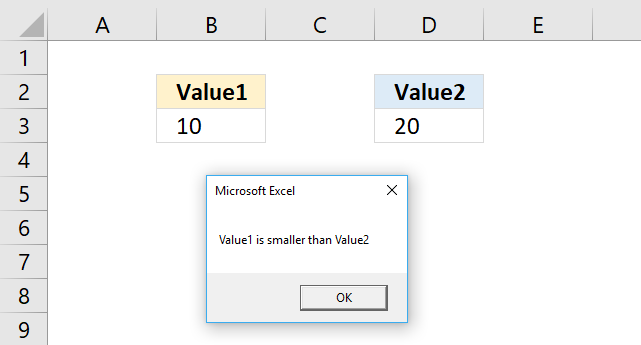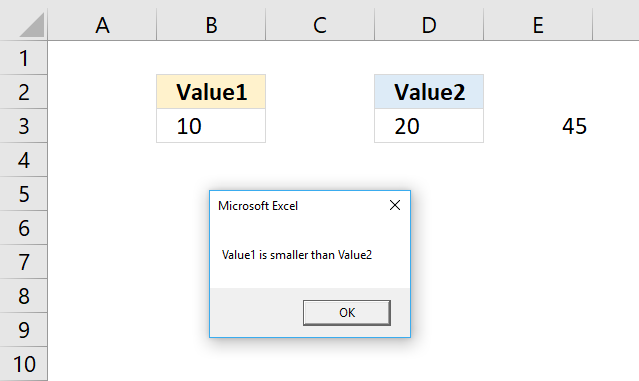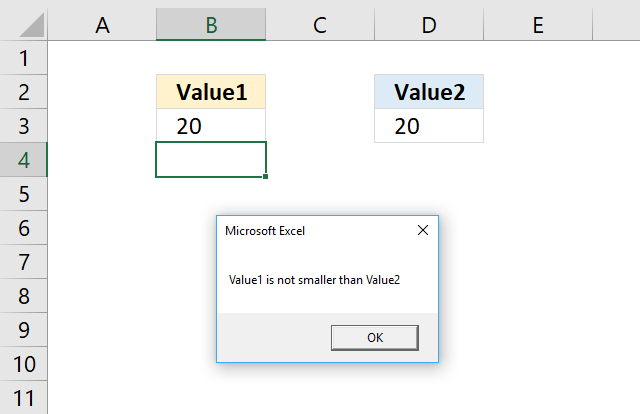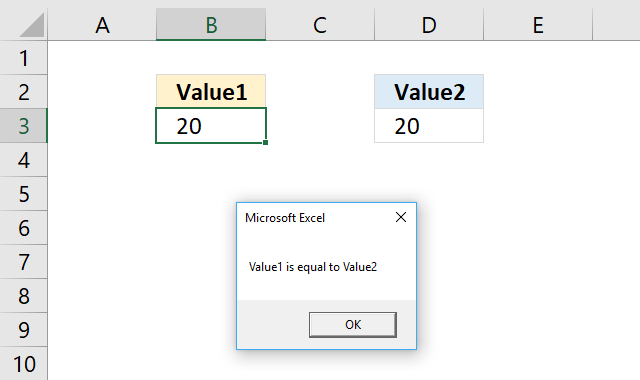Author: Oscar Cronquist Article last updated on January 15, 2018The picture above demonstrates an IF statement that checks if the value in B3 is smaller than the value in cell D3.

If true the If statement runs the remaining code after the Then statement, in this case, it shows a message box with text Value1 is smaller than Value2.

```Sub Macro1()

If Range("B3") < Range("D3") Then MsgBox "Value1 is smaller than Value2"

End Sub
```

### IF THEN END IFThe IF statement also allows you to run multiple lines of code, the END IF statement tells the subroutine when to stop.

```Sub Macro2()

If Range("B3") < Range("D3") Then

Range("E3") = 45

MsgBox "Value1 is smaller than Value2"

End if

End Sub
```

The subroutine above saves the number 45 to cell E3 if the value in cell B3 is smaller than the value in D3.

The msgbox function then displays Value1 is smaller than Value2.

### IF THEN ELSE ENDIFThe ELSE statement allows you to run code if the logical expression is not met.

```Sub Macro3()
If Range("B3") < Range("D3") Then
MsgBox "Value1 is smaller than Value2"
Else
MsgBox "Value1 is not smaller than Value2"
End If
End Sub
```

### IF THEN ELSEIF ELSE ENDIFThe ELSEIF statement lets you create another logical expression, you may have multiple ELSEIFs in the statement.

```Sub Macro4()
If Range("B3") < Range("D3") Then
MsgBox "Value1 is smaller than Value2"
ElseIf Range("B3") = Range("D3") Then
MsgBox "Value1 is equal to Value2"
Else
MsgBox "Value1 is larger than Value2"
End If
End Sub
```

### Get Excel *.xlsm macro-enabled file

IF THEN ELSE ENDIF.xlsm